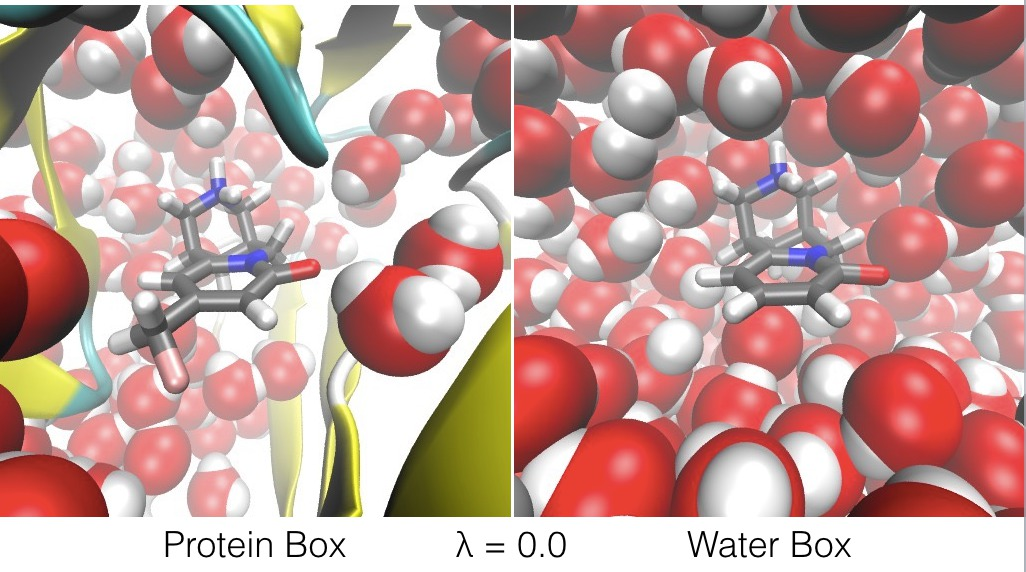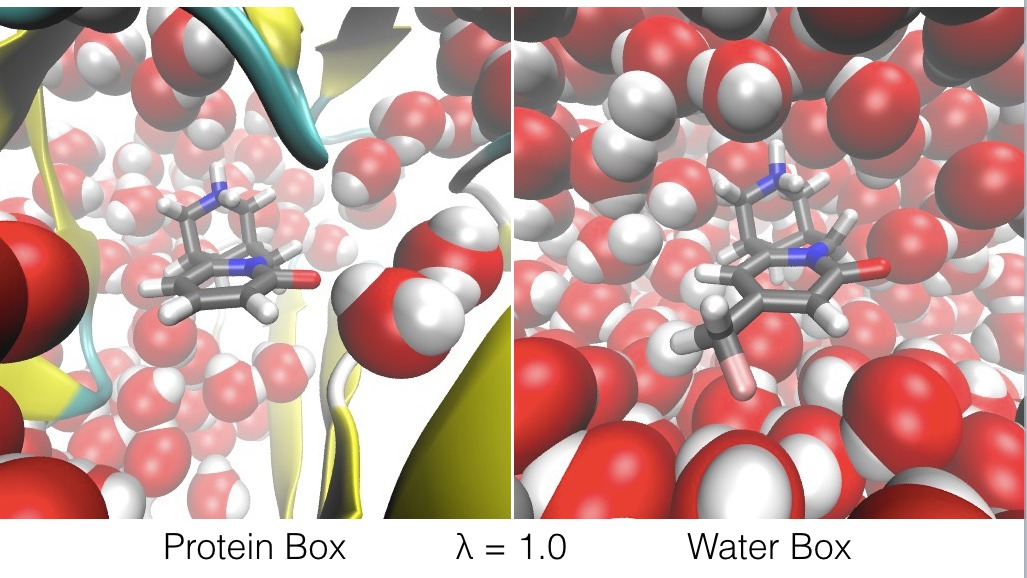Calculating relative binding free energies using ligandswap

This will be a short tutorial on how to use the ligandswap method implemented in the ligandswap program that comes with Sire to calculate relative binding free energies.

Ligandswap is an efficient and theoretically robust method of predicting the relative binding affinities of guest molecules (ligands) to host molecules (e.g. proteins).

For example, ligandswap can be used to calculate the relative binding free energy of two ligands, A and B, to a protein. It works by constructing a λ-coordinate that connects a periodic box of water containing ligand A bound to the protein (the Protein Box), with a second periodic box of water that contains ligand B on its own (the Water Box);The λ-coordinate is used to swap ligands A and B, such that;

• at λ=0.0, ligand A is bound to the protein, and ligand B is free in water (as shown above),
• at λ=1.0, ligand B is bound to the protein, and ligand A is free in water (as shown below),• and at λ values in between, ligands A and B are both partially bound to the protein, and both ligands A and B are both partially free in water (as shown below).The free energy change along this ligandswap λ reaction coordinate can be calculated using any standard free energy method, e.g. free energy perturbation (FEP), Bennett acceptance ratio method (BAR) or thermodynamic integration (TI). Indeed, to measure error, the ligandswap program with Sire calculates the free energy using all three methods at the same time.